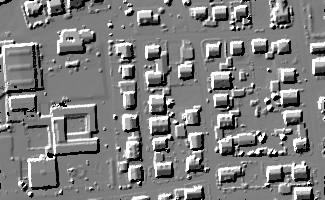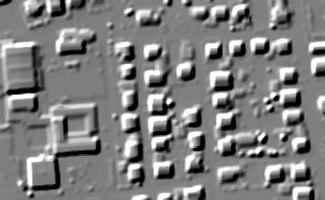Module Convolution

opals::IConvolution

# Aim of module

Performs convolutional filtering operations on a raster image.

# General Description

In the case of convolutional filtering, the filter kernel (parameter kernel, i.e. a raster image) is superimposed on the input raster (parameter inFile), and corresponding pixel values are multiplied and summed up. This sum-product value is the output value of the respective pixel. The kernel is shifted along the whole image, and convolution operation is repeated for each input raster pixel. The resulting output raster (parameter outFile) is stored in GDAL supported data format (parameter oFormat). The input and output raster structure is identical. However, the output raster may be restricted to a subset of the input rasters domain (parameter limit). Please note, that this feature is not yet implemented.

In general, the convolution filter requires a complete matrix of input pixels to be superimposed with the kernel matrix. This entails that pixel values beyond the image border have to be provided in order to process the edge pixels correctly. The following parameters are offered to estimate pixel values beyond the border:

• trend: The trend at the image border is continued (i.e. linear extrapolation beyond the edge)
• mirror: The trend at the image border is continued reversely (i.e. row 1 is copied row 99, row 2-> row 998, etc. Same procedure for edge columns)
• propagate: The pixel values at the image edge are propagated beyond the border (i.e.: row 99 = row 998 = ... = row 0. Same procedure for columns)
• nodata: All pixels beyond the image border are treated as void (no-data) pixels. This is the default. As a result, all convolution operations requiring a complete matrix of data pixels will fail at the image borders, resulting in an output raster being smaller than the original input raster by the size of the kernel.

In case there are void data pixels in the current neighbourhood, the sum-product can either be calculated by omitting these void pixels or the respective output pixel itself may be set to void (parameter ignoreNoData). Please note, that void pixels are generated if all image pixels within the current kernel area are invalid (i.e. nodata). Additionally, the marker indicating data voids in the output raster can be set by the user (parameter noData). Finally, the pixel-wise sum-product may be stored as is, or otherwise normalized, i.e. divided by the sum of the kernel values (parameter normalize).

# Kernel definition

The kernel matrix constitutes the main input for Module Convolution besides the input raster itself. Kernels can either be selected form a list of pre-defined kernels or specified explicitly by the user. The following syntax applies:

AVERAGE(ncols [nrows]) |
GAUSSIAN(SIZE(ncols [nrows]) SIGMA(stddev)) |
FROMFILE(imagefile) |
USERDEF(SIZE(ncols [nrows]) VALUES(k11 k12 ... ... ... knm ))

The first two (AVERAGE, GAUSSIAN) are pre-defined kernels requiring only the kernel size (number of columns/rows). The Gaussian kernel additionally requires the standard deviation of the gauss function (SIGMA).

Arbitrary user-defined kernels can be read from an existing raster file (FROMFILE) in GDAL supported data format. The georeferencing of the raster file is ignored, but the kernel matrix as read from file is superimposed to the image matrix 1:1. Note, that void pixels are not allowed in the kernel matrix. Finally, the kernel matrix (KM) can also be specified manually by providing both the size of the kernel (m..columns,n..rows) and the matrix elements themselves (elements in row major order, i.e.: k11 k12 ... knm)

$$\mathbf{KM} = \begin{pmatrix}k_{11} & k_{12} & k_{13} & ... & k_{1m} \\ k_{21} & k_{22} & k_{23} & ... & k_{2m} \\ ... & ... & ... & ... & ... \\ k_{n1} & k_{n2} & k_{n3} & ... & k_{nm} \end{pmatrix}$$,

Please note, that the rows and columns count both must be odd, for pre-defined as well as for user-defined kernels. The kernel matrix values are of float type.

## Examples:

AVERAGE(5)

A simple 5x5 average yielding the kernel matrix:

$$\mathbf{KM} = \begin{pmatrix} 1 & 1 & 1 & 1 & 1 \\ 1 & 1 & 1 & 1 & 1 \\ 1 & 1 & 1 & 1 & 1 \\ 1 & 1 & 1 & 1 & 1 \\ 1 & 1 & 1 & 1 & 1 \end{pmatrix}$$,

GAUSSIAN(SIZE(5 3) SIGMA(0.75))

An asymmetric 5 columns x 3 rows gaussian filter with a standard deviation of 0.75. The resulting kernel matrix is:

$$\mathbf{KM} = \begin{pmatrix} 0.0033 & 0.0478 & 0.1163 & 0.0478 & 0.0033 \\ 0.0081 & 0.1163 & 0.2829 & 0.1163 & 0.0081 \\ 0.0033 & 0.0478 & 0.1163 & 0.0478 & 0.0033 \\ \end{pmatrix}$$,

USERDEF(SIZE(3 3) VALUES( 1. 0. 1. 0. 1. 0. 1. 0. 1.))

A user defined 3x3 kernel. Note, the values are entered in row major order starting from the upper left corner, thus, the corresponding kernel matrix is:

$$\mathbf{KM} = \begin{pmatrix} 1.00 & 0.00 & 1.00\\ 0.00 & 1.00 & 0.00\\ 1.00 & 0.00 & 1.00\\ \end{pmatrix}$$,

FROMFILE(kernel.tif)

Finally, an example for reading the kernel matrix from a raster file (Tiff).

# Parameter description

-inFileinput raster file
Type: opals::Path
Remarks: mandatory
Input raster image in GDAL supported format,
-outFileoutput raster file
Type: opals::Path
Remarks: estimable
Path of convolved raster image file in GDAL supported format.
Estimation rule: The current directory and the name (body) of the input file is used as file name basis. Additionally, the postfix '_conv' and the extension corresponding to the output format are appended.
-oFormatraster file format [GTiff,AAIGrid,SCOP,...]
Type: opals::String
Remarks: estimable
Use GDAL driver names like GTiff, AAIGrid, USGSDEM, SCOP... .
Estimation rule: The output format is estimated based on the extension of the output file (*.tif->GTiff, *.dem->USGSDEM, *.dtm->SCOP...).
-kernelkernel type
Type: opals::ConvolutionKernel
Remarks: mandatory
Syntax for specifiying the convolution kernel matrix:
AVERAGE(ncols [nrows]) |
GAUSSIAN(SIZE(ncols [nrows]) SIGMA(stddev)) |
USERDEF(SIZE(ncols [nrows]) VALUES(k11 k12 ... ... ... knm ))
FROMFILE(imagefile)

-edgeHandlingedge handling method
Type: opals::EdgeHandling
Remarks: default=nodata
Possible values:
trend ....... Pixels beyond the image edge are linearly extrapolated based on the nearest and second nearest pixel value
mirror ...... Pixels beyond the image edge are mirrored, so the order of pixels is inverse.
propagate ... Pixels from the image border are propagated beyond the image edge.
nodata ...... Pixels beyond the image edge considered as nodata values. See noDataHandling parameter

For each pixel, convolution always takes the kernel environment into account. For the edge pixels it is, therefore, necessary to substitute the pixels outside the (input) raster with appropriate values from the image border. The following parameters are provided:
-ignoreNoDataignore no data values in neighbourhood
Type: bool
Remarks: default=1
If activated, all no-data pixels in the kernel neighbourhood are ignored in the convolution operation. If deactivated, the occurrence of a single no-data pixel in the kernel neighbourhood will result in a no-data pixel in the output.
-normalizenormalize result of convolution
Type: bool
Remarks: default=1
If activated, for each pixel the result of the convolution operation is normalized (i.e.: divided by the sum of the kernel values).
-limit2D clipping window
Type: opals::GridLimit
Remarks: optional
If no user defined limits are specified or -limit is even skipped, the entire xy-extents of the input raster model are used.
GridLimit: Defines xy-limits for output grid/raster datasets. The limit coordinates specified by left/lower/right/upper either refer to pixel centers (default) or corners, and may optionally get rounded to multiples of the grid size.
Syntax: ['center'|'corner']['round']['(' left lower right upper ')']
-noDatanoData value
Type: float
Remarks: default=9999
Value representing data voids int the output raster.
-gridSizegrid size value
Type: int32
Remarks: optional
Value defines the grid size according to the input kernel size

# Examples

The data used in the following example are located in the \$OPALS_ROOT/demo/ directory. For the strip 21 (contained in the demo directory) the following commands are required to obtain DSM model:

opalsImport -inf strip21.laz
opalsGrid -inf strip21.odm -outFile strip21.tif -inter movingPlanes

As a result, a grid file strip21.tif in GeoTiff format is created. A hill shading is shown in Figure 1.Figure 1: Hill shading of original DSM

## Example 1:

In this example smoothing is performed by applying a 5x5 Gaussian filter with a sigma value of 2.0.

opalsConvolution -infile strip21.tif -outfile strip21_gaussian_5x5.tif -kernel "GAUSSIAN(SIZE(5) SIGMA(2.0))"

A hill shading of the resulting model is shown in Figure 2.Figure 2: Smoothed DSM using a gaussian convolution kernel

## Example 2:

In this example smoothing is performed via a user-defined 3x3 filter. The calculated filter value is normalized with the number of non-noData values covered by the kernel.

opalsConvolution -infile strip21.tif -outfile strip21_smooth_3x3.tif -kernel "USERDEF(SIZE(3 3) VALUES(1 1 1 1 3 1 1 1 1))" -ignoreNoData 1 -normalize 1

## Example 3:

In this example a user-defined 3x3 filter in GDAL format is created and applied to a raster image.
It takes several steps to produce a filter kernel file in GDAL raster image format. One possible solution is as follows: Use a text editor, to create a xyz format ASCII file (e.g. conic_3x3.xyz in your working directory, containing the filter kernel coefficients. (For format definition see: Module Import).
conic_3x3.xyz should look like this:

1 1 0.1
2 1 0.2
3 1 0.1
1 0 0.2
2 0 0.3
3 0 0.2
1 99 0.1
2 99 0.2
3 99 0.1

(Use tab or blanks between numeric values.) First two coloumns denote the kernel matrix indices (columns and rows) while the third coloumn contains the kernel coefficients.
The first line corresspond to the top left corner of the 3x3 filter kernel (column 1, row 1), and the following lines correspond to the subsequent kernel coefficient (row major order). The last line corresponds to the bottom right corner of the filter kernel (column 3, row 99). The following commands are required to obtain the kernel in raster file format:

opalsImport -inf conic_3x3.xyz
opalsGrid -inf conic_3x3.odm -outFile conic_3x3.tif

The filter kernel file conic_3x3.tif in GeoTiff format is created and can, now, be used for convoluting a raster file:

opalsConvolution -infile strip21.tif -outfile strip21_smooth_3x3.tif -kernel "FROMFILE(conic_3x3.tif)"

# References

@ movingPlanes
moving (tilted) plane interpolation
opalsImport is the executable file of Module Import
Definition: ModuleExecutables.hpp:113
@ normalize
normalize results (e.g. opalsConvolution)
@ ignoreNoData
ignore no-data pixels withon kernel neighbourhood (opalsConvolution)
opalsGrid is the executable file of Module Grid
Definition: ModuleExecutables.hpp:93
opalsConvolution is the executable file of Module Convolution
Definition: ModuleExecutables.hpp:33